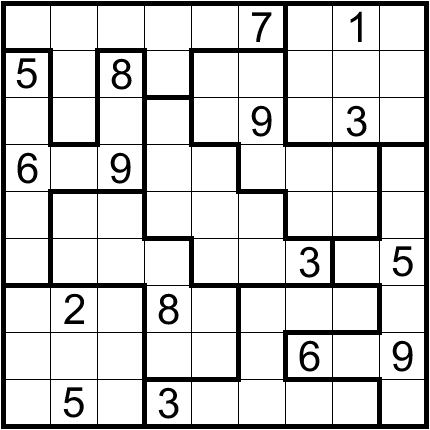Get inspired by the success stories of our students in IIT JAM MS, ISI  MStat, CMI MSc Data Science.  Learn More

# Non-Consecutive Selection | ISI MStat 2019 PSB Problem 3This problem is a beautiful and simple application of the bijection principle to count the number of non-consecutive selection of integers in combinatorics from Problem 3 of ISI MStat 2019 PSB.

## Problem - Non-Consecutive Selection

Elections are to be scheduled for any seven days in April and May. In how many ways can the seven days be chosen such that elections are not scheduled on two consecutive days?

### Prerequisites

• $a < b$, then $a$ and $b+1$ are never consecutive.
• Combination ( Choose Principle )
• Bijection Principle

## Solution

The problem is based on the first prerequisite mainly. That idea mathematicalizes the problem.

Out of the 61 days in April and May, we have to select 7 non-consecutive days. Let's convert this scenario to numbers.

Out of {$1, 2, 3, ... , 61$}, we have to select 7 non-consecutive numbers.

#### Lemma

$y_1 < y_2 < y_3 < ... < y_7$ are 7 non-consecutive numbers $\iff$ $y_i$ is of the form $x_i + (i-1)$ where $x_1 < x_2 < x_3 < ... < x_7$.

For example

You select {1, 3, 4, 5, 6, 8}. You change it to {1, 3 + 1, 4 + 2 , 5 + 3, 6 + 4 , 8 + 5} = { 1, 4, 6, 8, 10 , 13}, which are never consecutive.

Essentially, we are counting the non-consecutive integers in a different way, which helps us to count them.

So, we have to choose $x_1 < x_2 < x_3 < ... < x_7$, where the maximum $x_7 + (7-1) = x_7 + 6 \leq 61 \Rightarrow x_7 \leq 55$.

Hence, the problem boiled down to choosing $x_1 < x_2 < x_3 < ... < x_7$ from {$1, 2, 3, ... , 55$}, which is a combination problem.

We can have to just choose 7 such numbers. The number of ways to do so is ${55}\choose{7}$.

This problem is a beautiful and simple application of the bijection principle to count the number of non-consecutive selection of integers in combinatorics from Problem 3 of ISI MStat 2019 PSB.

## Problem - Non-Consecutive Selection

Elections are to be scheduled for any seven days in April and May. In how many ways can the seven days be chosen such that elections are not scheduled on two consecutive days?

### Prerequisites

• $a < b$, then $a$ and $b+1$ are never consecutive.
• Combination ( Choose Principle )
• Bijection Principle

## Solution

The problem is based on the first prerequisite mainly. That idea mathematicalizes the problem.

Out of the 61 days in April and May, we have to select 7 non-consecutive days. Let's convert this scenario to numbers.

Out of {$1, 2, 3, ... , 61$}, we have to select 7 non-consecutive numbers.

#### Lemma

$y_1 < y_2 < y_3 < ... < y_7$ are 7 non-consecutive numbers $\iff$ $y_i$ is of the form $x_i + (i-1)$ where $x_1 < x_2 < x_3 < ... < x_7$.

For example

You select {1, 3, 4, 5, 6, 8}. You change it to {1, 3 + 1, 4 + 2 , 5 + 3, 6 + 4 , 8 + 5} = { 1, 4, 6, 8, 10 , 13}, which are never consecutive.

Essentially, we are counting the non-consecutive integers in a different way, which helps us to count them.

So, we have to choose $x_1 < x_2 < x_3 < ... < x_7$, where the maximum $x_7 + (7-1) = x_7 + 6 \leq 61 \Rightarrow x_7 \leq 55$.

Hence, the problem boiled down to choosing $x_1 < x_2 < x_3 < ... < x_7$ from {$1, 2, 3, ... , 55$}, which is a combination problem.

We can have to just choose 7 such numbers. The number of ways to do so is ${55}\choose{7}$.

This site uses Akismet to reduce spam. Learn how your comment data is processed.

### Knowledge Partner

Cheenta is a knowledge partner of Aditya Birla Education Academy